Saturday, June 12, 2021
Home > Latest Announcement > NTSE 2018-19 Stage 2 Question Paper With Answer Keys & Solutions

# NTSE 2018-19 Stage 2 Question Paper With Answer Keys & Solutions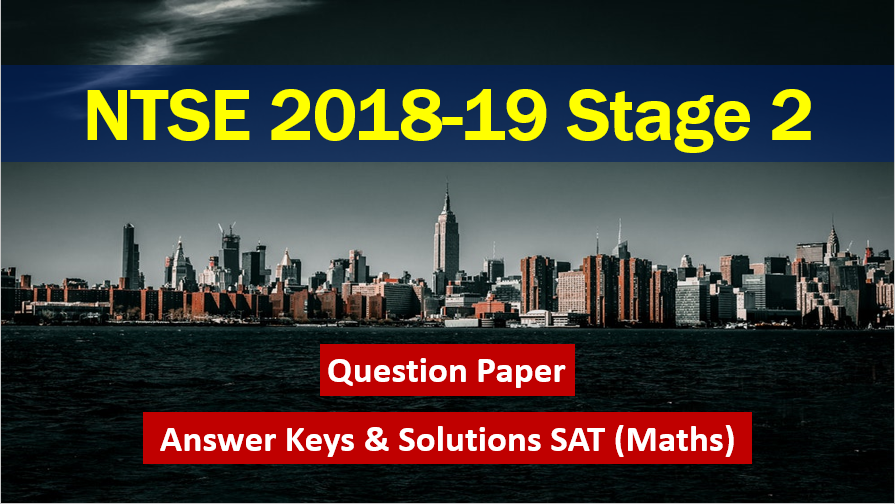# NTSE 2018-19 Stage 2 Question Paper With Answer Keys

Hi Students, welcome to Amans Maths Blogs (AMB). On this page, you will get NTSE 2018 Stage 2 Question Paper With Answer Keys and SAT (Maths) Solutions.

As we know that NTSE 2018-19 is a national level program  which is conducted in two stages NTSE 2018 Stage 1 which was scheduled on 04 November 2018, conducted by their respective states and union territories and NTSE 2018 Stage 2 is conducted by NCERT (National Council of Educational Research and Training).

NTSE Stage 1 and 2 is the one of the most prestigious exams in India. To know more about NTSE like NTSE notification, syllabus, exam pattern, FAQ, previous year question papers with solution, click here

On this post, you will get the NTSE 2019 Stage 2 Question Paper & Answer Keys and Solutions. Here, you will get NTSE 2019 Stage 2 SAT (Maths) Solutions. These questions are solved by me, AMAN RAJ, I am maths faculty of IIT Maths Foundation course and founder of this website AmansMathsBlogs (AMB).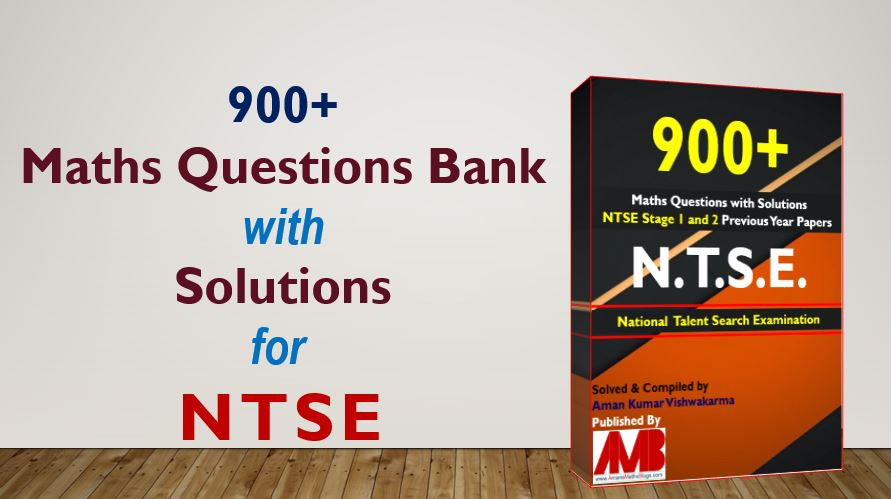## NTSE 2018-19 Stage 2 MAT Question Paper

This file will be uploaded soon…

## NTSE 2018-19 Stage 2 MAT Answer Keys

NTSE 2018-9 Stage 2 Answer Keys
NTSE 2019 Stage 2 MAT Answer Keys
Ques NoAns KeyQues NoAns KeyQues NoAns KeyQues NoAns Key
11261513762
21271524773
32282533783
41294542792
52302554803
62312561814
73321572823
83333583832
92344591844
102353603853
113363613862
124372623873
132383633882
141393642893
154402653902
163412662912
173424672923
183432683932
194442693944
201453701953
212461713964
224474722974
231481731984
24249NA742991
2525027541001

## NTSE 2018-19 Stage 2 SAT Answer Keys

Social Science (1 to 40)
Social Science NTSE 2019 Stage 2 Answer Keys
Ques NoAns KeyQues NoAns KeyQues NoAns KeyQues NoAns Key
13112212314
23123224322
34132232332
43143243342
54152254354
62161263364
72173273371
81183281384
93192294394
101201302404
Mathematics (41 to 60)
Maths NTSE 2018-19 Stage 2 Answer Keys
Ques NoAns KeyQues NoAns KeyQues NoAns KeyQues NoAns Key
412461512563
424473521573
431483532582
441493542592
451504553601
Physics (61 to 73)
PHYSICS NTSE 2018-19 Stage 2 Answer Keys
Ques NoAns KeyQues NoAns KeyQues NoAns KeyQues NoAns Key
613664711
623672723
632684732
64NA691
652702
Chemistry (74 to 87)
CHEMISTRY NTSE 2018-19 Stage 2 Answer Keys
Ques NoAns KeyQues NoAns KeyQues NoAns KeyQues NoAns Key
744791843
751802853
763814864
773823873
782834
Biology (88 to 100)
BIOLOGY NTSE 2018-19 Stage 2 Answer Keys
Ques NoAns KeyQues NoAns KeyQues NoAns KeyQues NoAns Key
883932991
8929531002
903963
911973
921983

## NTSE Maths Combo Pack EBook

Get 900+ NTSE Maths Previous Year Question Bank with Solutions and 11 Mock Test Papers EBook for NTSE exams. For more details, Click To NTSE Stage 1 Mock Test SAT Maths and NTSE Stage 2 Mock Test SAT Maths. To get this combo ebook, click below RED button.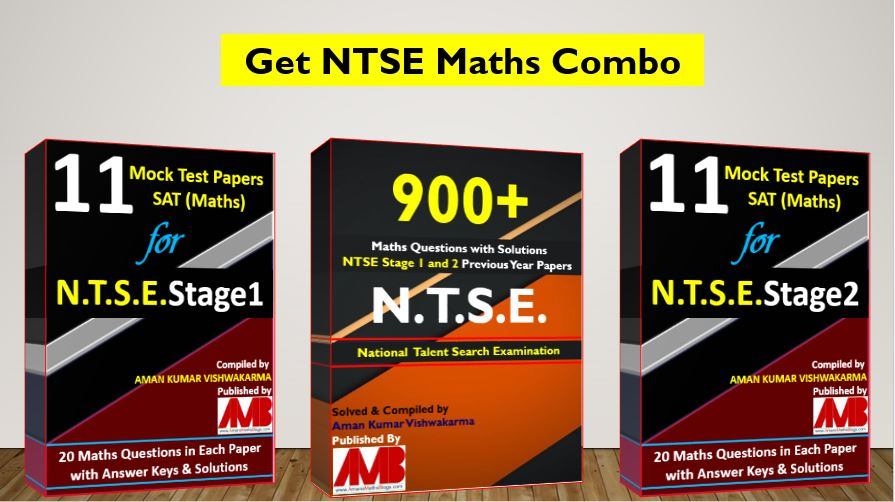900+ Math Questions Bank & 11 Mock Test Paper for NTSE Stage 1 & 2 SAT (Maths)

## NTSE 2018-19 Stage 2 SAT (Maths) Solutions

NTSE 2019 Stage 2 SAT Math Solutions: Ques No 41

If m = n2 – n, where n is an integer, then m2 – 2m is divisible by

Options:

1. 20

2. 24

3. 30

4. 16

Answer Key: 2

Solution: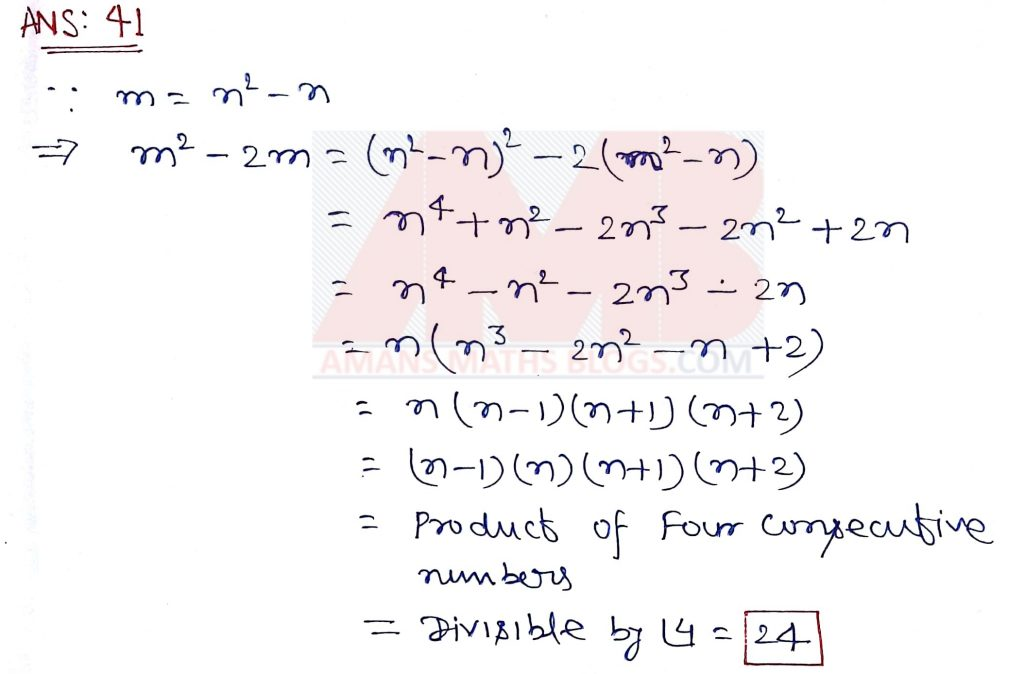NTSE 2019 Stage 2 SAT Math Solutions: Ques No 42

The value of √[(97 x 98 x 99 x 100) + 1] is equal to

Options:

1. 9901

2. 9891

3. 9801

4. 9701

Answer Key: 4

Solution:NTSE 2019 Stage 2 SAT Math Solutions: Ques No 43

Let P(x) be a polynomial of degree 3 and P(n) = 1/n for n = 1, 2, 3, 4. Then, the value of P(5) is

Options:

1. 0

2. 1/5

3. -2/5

4. 3/5

Answer Key: 1

Solution:NTSE 2019 Stage 2 SAT Math Solutions: Ques No 44

If α and β are the roots of the equation 3x2 – 5x + 3 = 0, then the quadratic equation whose he roots are α2β and αβ2 is

Options:

1. 3x2 – 5x + 3 = 0

2. 3x2 – 8x + 3 = 0

3. 3x2 – 8x + 3 = 0

4. 3x2 – 5x – 3 = 0

Answer Key: 1

Solution:NTSE 2019 Stage 2 SAT Math Solutions: Ques No 45

In village Madhubani 8 women and 12 girls can paint a large mural in 10 hours. 6 women and 8 girls can paint it in 14 hours. The number of hours taken by 7 women and 14 girls to pain the mural is

Options:

1. 10

2. 15

3. 20

4. 35

Answer Key: 1

Solution: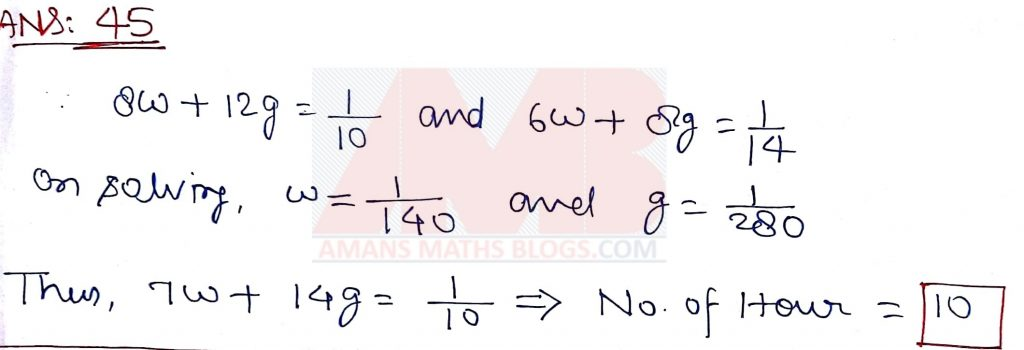NTSE 2019 Stage 2 SAT Math Solutions: Ques No 46

If x = (3 + √5) / 2 and y = x3, then y satisfies the quadratic equation

Options:

1. y2 – 18y + 1 = 0

2. y2 + 18y + 1 = 0

3. y2 – 18y – 1 = 0

4. y2 + 18y – 1 = 0

Answer Key: 1

Solution:NTSE 2019 Stage 2 SAT Math Solutions: Ques No 47

If tan2θ = 1 – e2, then the value of secθ + tan3θcosecθ is equal to

Options:

1. (1 – e2)1/2

2. (2 – e2)1/2

3. (2 – e2)3/2

4. (1 – e2)3/2

Answer Key: 3

Solution: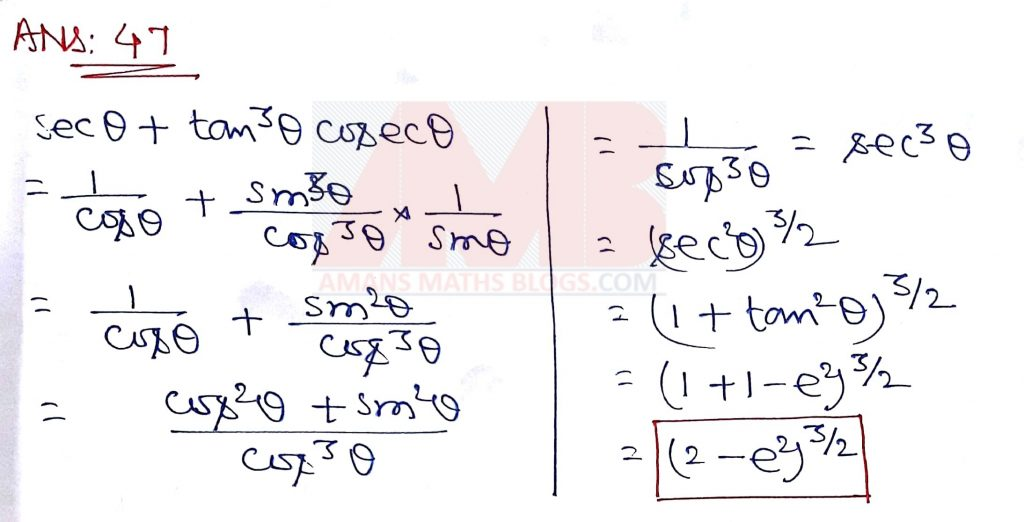NTSE 2019 Stage 2 SAT Math Solutions: Ques No 48

Let the volume of a solid sphere be 288π cm3. A horizontal plane cuts the sphere at a distance of 3 cm from the centre so that the ratio of the curved surface areas of the two parts of the sphere is 3 : 1. The total surface area of the bigger part of the sphere (in cm2) is

Options:

1. 36π

2. 108π

3. 135π

4. 144π

Answer Key: 2

Solution: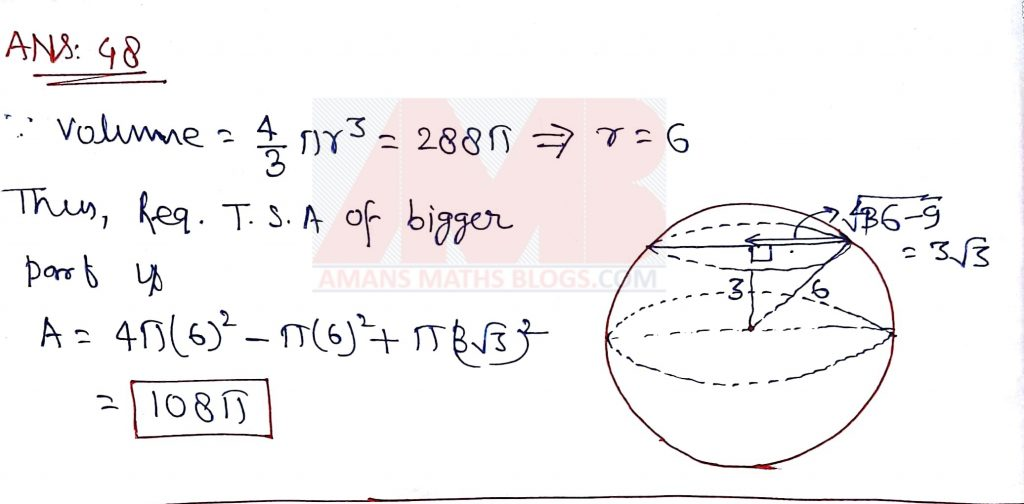NTSE 2019 Stage 2 SAT Math Solutions: Ques No 49

A solid metallic cylinder of height 10 cm and diameter 14 cm is melted to make two cones in the proportion of their volumes as 3 : 4, keeping the height 10 cm, what would be the percentage increase in the flat surface area?

Options:

1. 9

2. 16

3. 50

4. 200

Answer Key: 3

Solution:NTSE 2019 Stage 2 SAT Math Solutions: Ques No 50

Each vertical face of square based vertical pillar of height 3 m has 7 equal, semi-cylindrical surfaces in such a way that its horizontal cross-section is as shown in the figure. If the radius of each pillar so designed (taking π = 22/7) isOptions:

1. 5.88

2. 6.14

3. 6.42

4. 7.2

Answer Key: 4

Solution:NTSE 2019 Stage 2 SAT Math Solutions: Ques No 51

Let ABCD be a square of side 20 cm. The area of square PQRS (in cm2) interior to ABCD, shown in the figure is.Options:

1. 60

2. 80

3. 100

4. 400

Answer Key: 2

Solution:NTSE 2019 Stage 2 SAT Math Solutions: Ques No 52

A circle is inscribed in a right angled triangle of perimeter 7π. Then the ratio of numerical values of circumference of the circle to the area of the right angled triangle is

Options:

1. 4 : 7

2. 3 : 7

3. 2 : 7

4. 1 : 7

Answer Key: 1

Solution: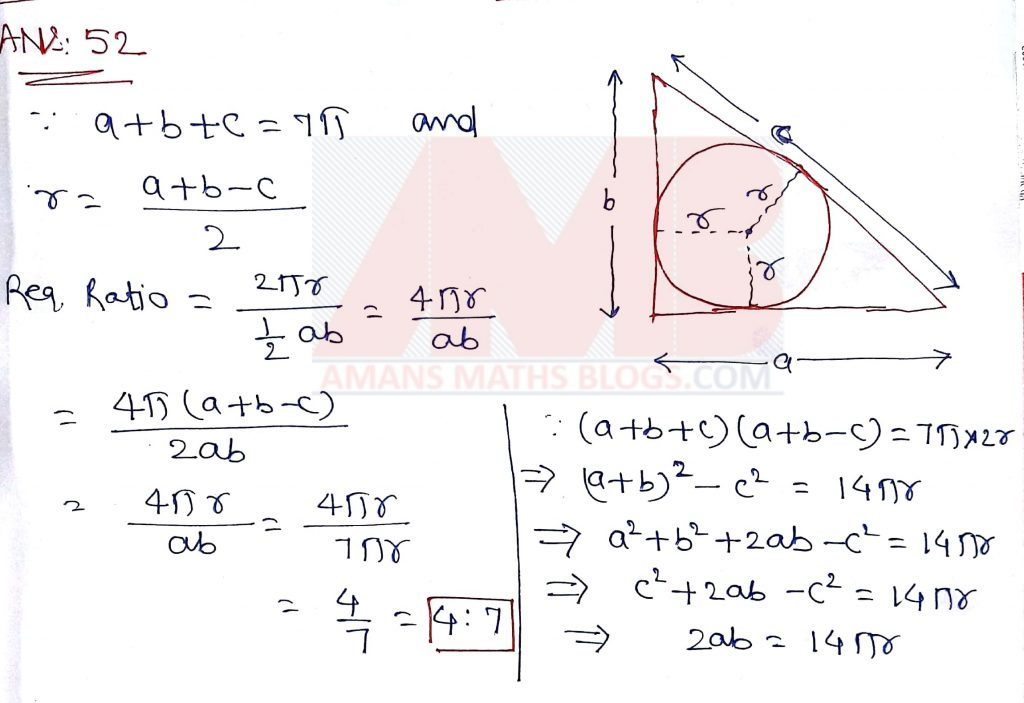NTSE 2019 Stage 2 SAT Math Solutions: Ques No 53

It is known that the area of cyclic quadrilateral is √(S – a)(S – b)(S – c)(S – d), where a, b, c and d are the sides and S = (a + b + c + d)/2. If a circle can also be the inscribed in the cyclic quadrilateral is

Options:

1. √( (ab)2 + (cd)2)

2. √(abcd)

3. √( (ac)2 + (bd)2)

4. √( (ad)2 + (bc)2)

Answer Key: 2

Solution:NTSE 2019 Stage 2 SAT Math Solutions: Ques No 54

Two circles both of radii a touch each other and each of them touches internally a circle of radius 2a. Then the radius of the circle which touches all the three circles is

Options:

1. a/2

2. 2a/3

3. 3a/4

4. a

Answer Key: 2

Solution: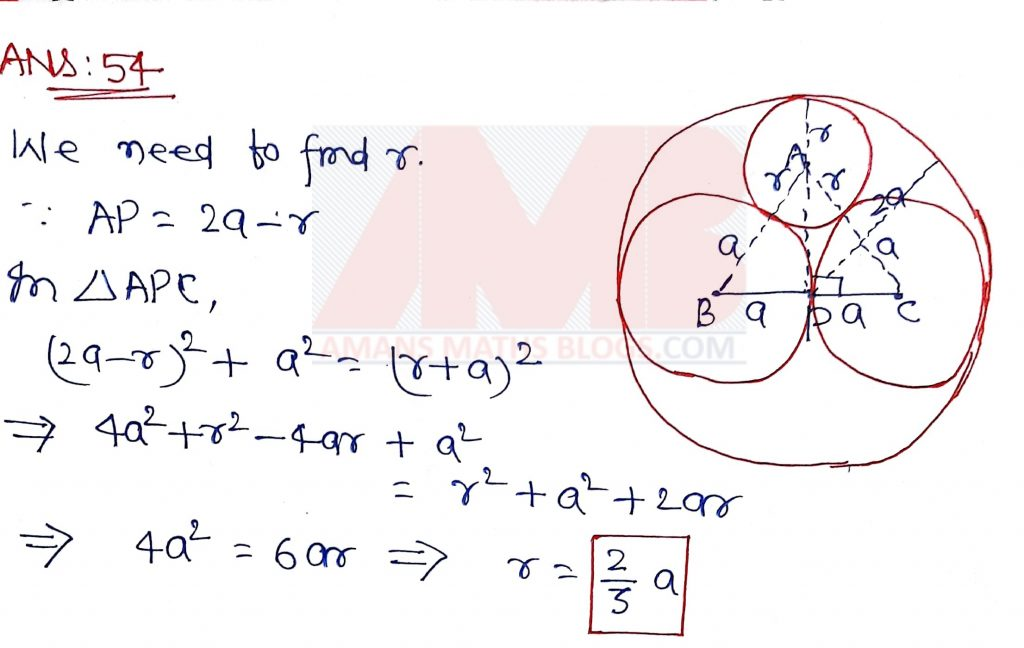NTSE 2019 Stage 2 SAT Math Solutions: Ques No 55

Let D be a point on the side BC of a triangle ABC such that ∠ ADC = ∠BAC. If AC = 21 cm, then the side of an equilateral triangle whose area is equal to the area of the rectangle with sides BC and DC is

Options:

1. 14 x 31/2

2. 42 x 3-1/2

3. 14 x 33/2

4. 42 x 31/2

Answer Key: 3

Solution:NTSE 2019 Stage 2 SAT Math Solutions: Ques No 56

Let ABC be a triangle with sides a, b, c. Then, the length of medians of the triangle formed by the medians of the triangle ABC are

Options:

1. a/2, b/2, c/2

2. 2a/3, 2b/3, 2c/3

3. 3a/4, 3b/4, 3c/4

4. 5a/6, 5b/6, 5c/6

Answer Key: 3

Solution: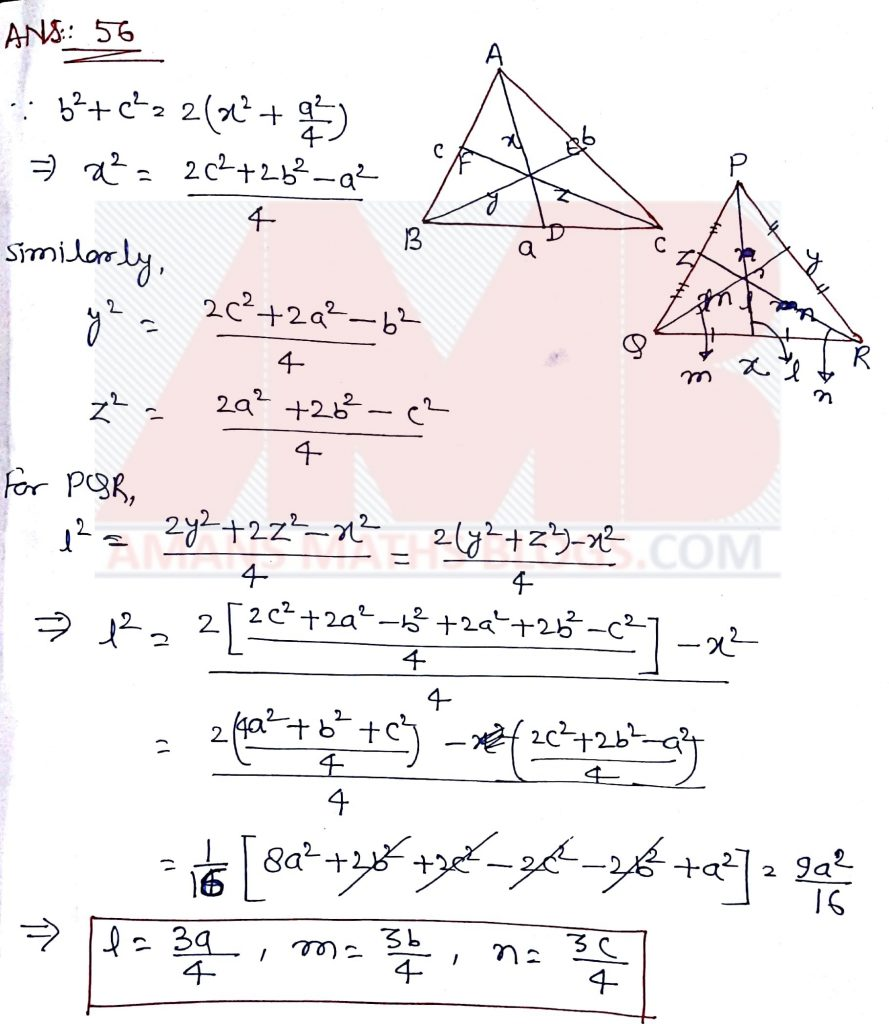NTSE 2019 Stage 2 SAT Math Solutions: Ques No 57

(x + 1)4 is divided by (x – 1)3. Then the value of the remainder at x = 1 is

Options:

1. -16

2. 0

3. 16

4. 32

Answer Key: 3

Solution:NTSE 2019 Stage 2 SAT Math Solutions: Ques No 58

A circle passes through the vertices of triangle ABC. If the vertices are A(-2, 5), B(-2, -3), C(2, -3), then the center of the circle is

Options:

1. (0, 0)

2. (0, 1)

3. (-2, 1)

4. (0, -3)

Answer Key: 2

Solution:NTSE 2019 Stage 2 SAT Math Solutions: Ques No 59

If two dice are thrown together, the probability that the difference of the numbers appearing on them is a prime number is

Options:

1. 2/9

2. 4/9

3. 5/12

4. 17/36

Answer Key: 2

Solution:NTSE 2019 Stage 2 SAT Math Solutions: Ques No 60

Observe the following data.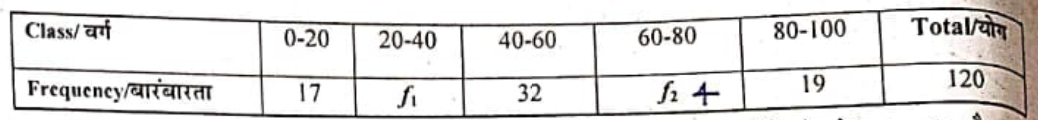If the above data has mean 50, then the missing frequencies f1 and f2 are respectively.

Options:

1. 28 and 24

2. 24 and 28

3. 28 and 30

4. 30 and 28

Answer Key: 1

Solution:## NTSE Maths Combo Pack EBook

Get 900+ NTSE Maths Previous Year Question Bank with Solutions and 11 Mock Test Papers EBook for NTSE exams. For more details, Click To NTSE Stage 1 Mock Test SAT Maths and NTSE Stage 2 Mock Test SAT Maths. To get this combo ebook, click below RED button.900+ Math Questions Bank & 11 Mock Test Paper for NTSE Stage 1 & 2 SAT (Maths)

error: Content is protected !!